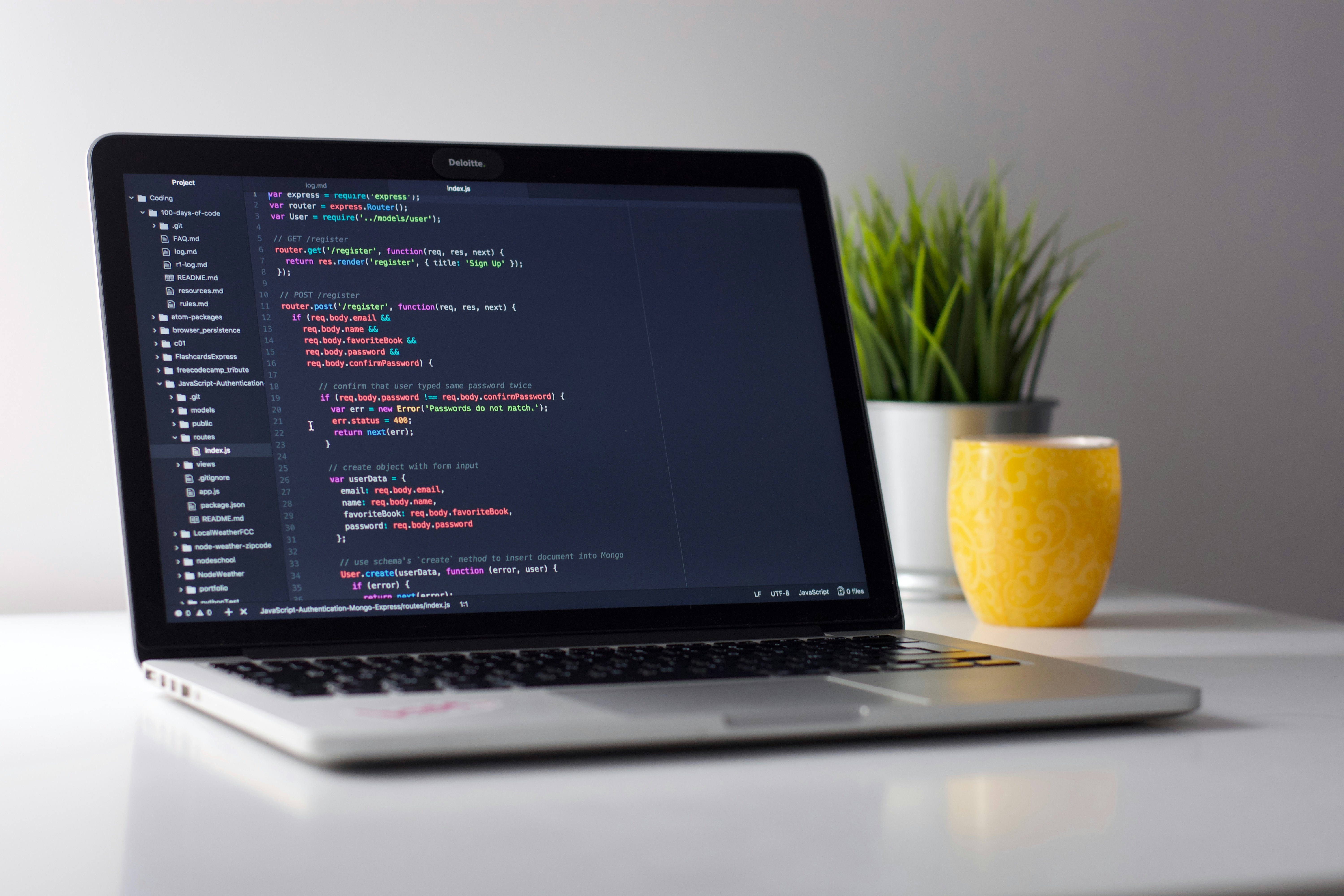type
status
date
slug
summary
tags
category
icon
Property
Apr 23, 2023 06:19 AM

#### 变量和类型

• 整型：Python中可以处理任意大小的整数（Python 2.x中有`int``long`两种类型的整数，但这种区分对Python来说意义不大，因此在Python 3.x中整数只有int这一种了），而且支持二进制（如`0b100`，换算成十进制是4）、八进制（如`0o100`，换算成十进制是64）、十进制（`100`）和十六进制（`0x100`，换算成十进制是256）的表示法。
• 浮点型：浮点数也就是小数，之所以称为浮点数，是因为按照科学记数法表示时，一个浮点数的小数点位置是可变的，浮点数除了数学写法（如`123.456`）之外还支持科学计数法（如`1.23456e2`）。
• 字符串型：字符串是以单引号或双引号括起来的任意文本，比如`'hello'``"hello"`,字符串还有原始字符串表示法、字节字符串表示法、Unicode字符串表示法，而且可以书写成多行的形式（用三个单引号或三个双引号开头，三个单引号或三个双引号结尾）。
• 布尔型：布尔值只有`True``False`两种值，要么是`True`，要么是`False`，在Python中，可以直接用`True``False`表示布尔值（请注意大小写），也可以通过布尔运算计算出来（例如`3 < 5`会产生布尔值`True`，而`2 == 1`会产生布尔值`False`）。
• 复数型：形如`3+5j`，跟数学上的复数表示一样，唯一不同的是虚部的`i`换成了`j`。实际上，这个类型并不常用，大家了解一下就可以了。

#### 变量命名

• 硬性规则：
• 变量名由字母（广义的Unicode字符，不包括特殊字符）、数字和下划线构成，数字不能开头。
• 大小写敏感（大写的`a`和小写的`A`是两个不同的变量）。
• 不要跟关键字（有特殊含义的单词，后面会讲到）和系统保留字（如函数、模块等的名字）冲突。
• PEP 8要求：
• 用小写字母拼写，多个单词用下划线连接。
• 受保护的实例属性用单个下划线开头（后面会讲到）。
• 私有的实例属性用两个下划线开头（后面会讲到）。

#### 变量的使用

```""" 使用变量保存数据并进行加减乘除运算 Version: 0.1 Author: 骆昊 """ a = 321 b = 12 print(a + b) # 333 print(a - b) # 309 print(a * b) # 3852 print(a / b) # 26.75```

```""" 使用type()检查变量的类型 Version: 0.1 Author: 骆昊 """ a = 100 b = 12.345 c = 1 + 5j d = 'hello, world' e = True print(type(a)) # <class 'int'> print(type(b)) # <class 'float'> print(type(c)) # <class 'complex'> print(type(d)) # <class 'str'> print(type(e)) # <class 'bool'>```

• `int()`：将一个数值或字符串转换成整数，可以指定进制。
• `float()`：将一个字符串转换成浮点数。
• `str()`：将指定的对象转换成字符串形式，可以指定编码。
• `chr()`：将整数转换成该编码对应的字符串（一个字符）。
• `ord()`：将字符串（一个字符）转换成对应的编码（整数）。

```""" 使用input()函数获取键盘输入(字符串) 使用int()函数将输入的字符串转换成整数 使用print()函数输出带占位符的字符串 Version: 0.1 Author: 骆昊 """ a = int(input('a = ')) b = int(input('b = ')) print('%d + %d = %d' % (a, b, a + b)) print('%d - %d = %d' % (a, b, a - b)) print('%d * %d = %d' % (a, b, a * b)) print('%d / %d = %f' % (a, b, a / b)) print('%d // %d = %d' % (a, b, a // b)) print('%d %% %d = %d' % (a, b, a % b)) print('%d ** %d = %d' % (a, b, a ** b))```

#### 运算符

Python支持多种运算符，下表大致按照优先级从高到低的顺序列出了所有的运算符，运算符的优先级指的是多个运算符同时出现时，先做什么运算然后再做什么运算。除了我们之前已经用过的赋值运算符和算术运算符，我们稍后会陆续讲到其他运算符的使用。
 运算符 描述 `[]` `[:]` 下标，切片 `**` 指数 `~` `+` `-` 按位取反, 正负号 `*` `/` `%` `//` 乘，除，模，整除 `+` `-` 加，减 `>>` `<<` 右移，左移 `&` 按位与 `^` `|` 按位异或，按位或 `<=` `<` `>` `>=` 小于等于，小于，大于，大于等于 `==` `!=` 等于，不等于 `is` `is not` 身份运算符 `in` `not in` 成员运算符 `not` `or` `and` 逻辑运算符 `=` `+=` `-=` `*=` `/=` `%=` `//=` `**=` `&=` ` =` `^=` `>>=` `<<=`

#### 赋值运算符

```""" 赋值运算符和复合赋值运算符 Version: 0.1 Author: 骆昊 """ a = 10 b = 3 a += b # 相当于：a = a + b a *= a + 2 # 相当于：a = a * (a + 2) print(a) # 算一下这里会输出什么```

#### 比较运算符和逻辑运算符

```""" 比较运算符和逻辑运算符的使用 Version: 0.1 Author: 骆昊 """ flag0 = 1 == 1 flag1 = 3 > 2 flag2 = 2 < 1 flag3 = flag1 and flag2 flag4 = flag1 or flag2 flag5 = not (1 != 2) print('flag0 =', flag0) # flag0 = True print('flag1 =', flag1) # flag1 = True print('flag2 =', flag2) # flag2 = False print('flag3 =', flag3) # flag3 = False print('flag4 =', flag4) # flag4 = True print('flag5 =', flag5) # flag5 = False```

#### 练习1：华氏温度转换为摄氏温度。

```""" 将华氏温度转换为摄氏温度 Version: 0.1 Author: 骆昊 """ f = float(input('请输入华氏温度: ')) c = (f - 32) / 1.8 print('%.1f华氏度 = %.1f摄氏度' % (f, c))```

`print(f'{f:.1f}华氏度 = {c:.1f}摄氏度')`

#### 练习2：输入圆的半径计算计算周长和面积。

```""" 输入半径计算圆的周长和面积 Version: 0.1 Author: 骆昊 """ radius = float(input('请输入圆的半径: ')) perimeter = 2 * 3.1416 * radius area = 3.1416 * radius * radius print('周长: %.2f' % perimeter) print('面积: %.2f' % area)```

#### 练习3：输入年份判断是不是闰年。

``````"""

Version: 0.1
Author: 骆昊
"""
year = int(input('请输入年份: '))
# 如果代码太长写成一行不便于阅读 可以使用\对代码进行折行
is_leap = year % 4 == 0 and year % 100 != 0 or \
year % 400 == 0
print(is_leap)``````

• Waline
• ValineIOS付费应用推荐
2023-4-25PRD：注册登录功能文档
2023-4-24Python100天-04循环结构
2023-4-24Python100天-03分支结构
2023-4-23Python100天-02语言元素
2023-4-23

type
status
date
slug
summary
tags
category
icon
``ChatOpen.xyz``